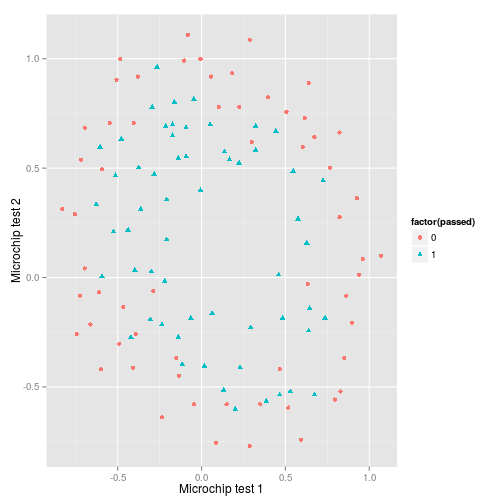### Matt Upson

Yo no soy marinero

# Non-linear classification with logistic regression

In my last post I compared vectorised logistic regression solved with an optimisation algorithm with a generalised linear model. I tested it out on a very simple dataset which could be classified using a linear boundary. In this post I’m following the next part of Andrew Ng’s Machine Learning course on coursera and implementing regularisation and feature mapping to allow me to map non-linear decision boundaries using logistic regression. And of course, I’m doing it in R, not Matlab or Octave.

As ever the full code to produce this page is available on github.

### Visualising the data

First I plot the data…and it’s pretty clear that to create an accurate decision boundary will require some degree of polynomial features in order to account for its spherical nature.### Feature mapping

In this example I’ll map the features into all polynomial terms of $x_1$ and $x_2$ up to the twelfth power giving a crazy amount of input features. Hence:

$mF(x)=\begin{bmatrix} 1 \\ x_1 \\ x_2 \\ x_1^2 \\ x_1 x_2 \\ x_2^2 \\ x_1^3 \\ \vdots \\ x_1x_2^{11} \\ x_2^{12} \end{bmatrix}$

These polynomials can be calculated with the following code. In future I will update this to take more than two input features.

And to the list the 91 features:

Now run the linear regression I implemented in my previous post.